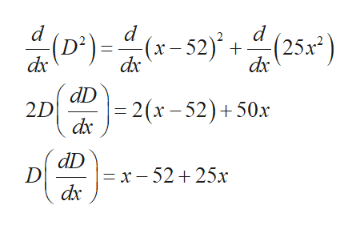# Find the point P on the line y = 5x that is closest to the point (52,0).  What is the least distance between P and (52,0)?Let D be the distance between the two points. What is the objective function in terms of one number, x?D =

Question
354 views

Find the point P on the line y = 5x that is closest to the point (52,0).  What is the least distance between P and (52,0)?

Let D be the distance between the two points. What is the objective function in terms of one number, x?

D =

check_circle

Step 1

Given:

The equation of the line is y = 5x.

(52,0) is the closest point to the point P which line on the line y= 5x.

Step 2

Calculation:

Let P(x,5x) and the other point be (52,0).

Compute the distance between the points P(x,5x) and (52,0) as follows.

Step 3

Now, derivative the above obtained distance equation ...help_outlineImage Transcriptionclose(D)=-52)+(25x") d d d (х-52)* +- dx dD 2D | 2 ( x-52)+ 50x dx dD D =x-52+ 25x dx fullscreen

### Want to see the full answer?

See Solution

#### Want to see this answer and more?

Solutions are written by subject experts who are available 24/7. Questions are typically answered within 1 hour.*

See Solution
*Response times may vary by subject and question.
Tagged in

### Other